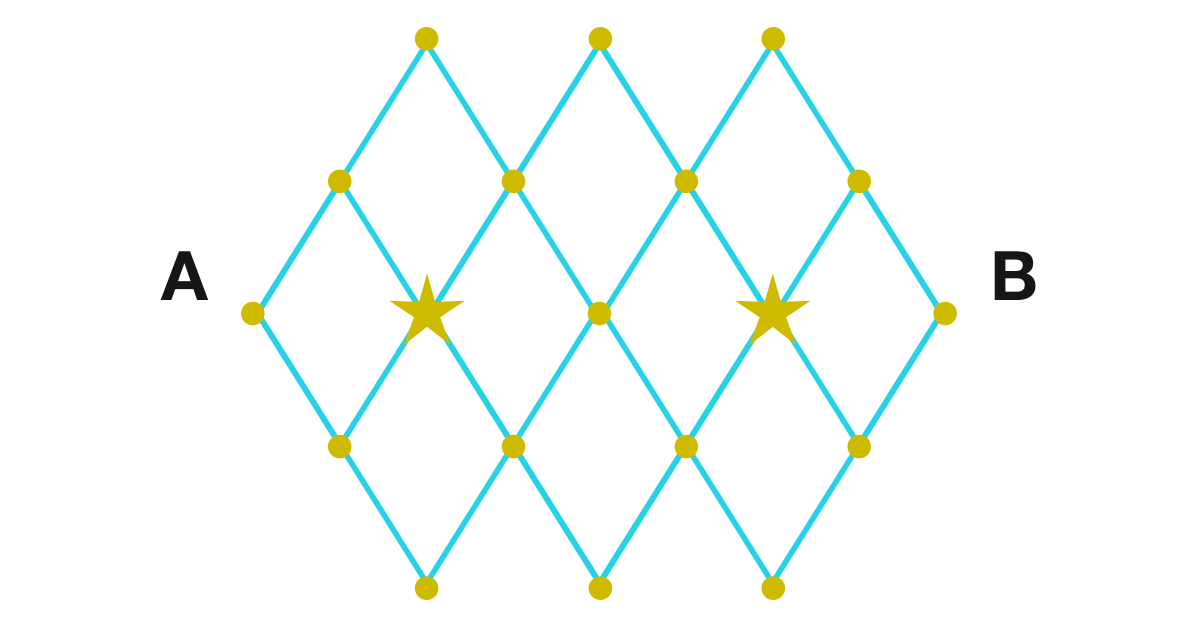# Rhombus Grid

Probability Level 3

To go from $A$ to $B$, you only can move along $\nearrow$ and $\searrow$ and you can't pass through more than one star.How many paths are there to go from $A$ to $B$?

×